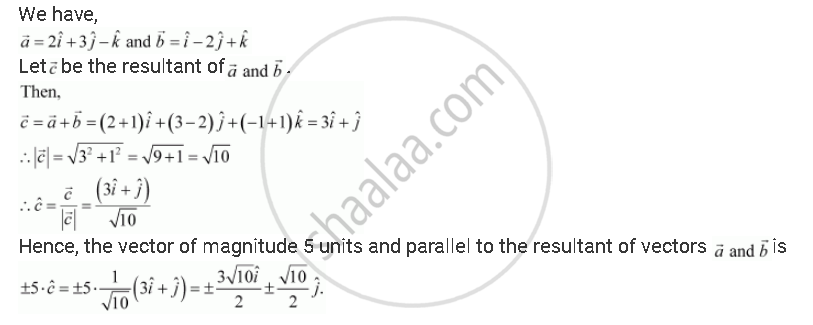Share

# Find a Vector of Magnitude 5 Units, and Parallel to the Resultant of the Vectors - CBSE (Science) Class 12 - Mathematics

ConceptMagnitude and Direction of a Vector

#### Question

Find a vector of magnitude 5 units, and parallel to the resultant of the vectors veca = 2i + 3hatj - hatk and vecb = hati - 2hatj + hatk

#### SolutionIs there an error in this question or solution?

#### APPEARS IN

Solution Find a Vector of Magnitude 5 Units, and Parallel to the Resultant of the Vectors Concept: Magnitude and Direction of a Vector.
S